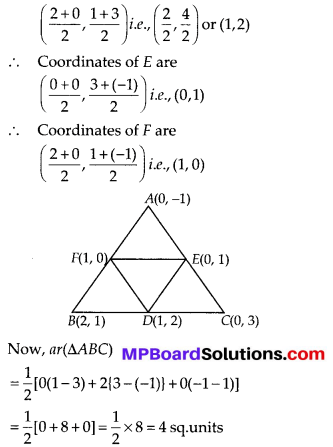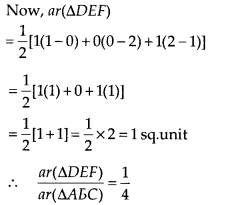# MP Board Class 10th Maths Solutions Chapter 7 Coordinate Geometry Ex 7.3

In this article, we will share MP Board Class 10th Maths Book Solutions Chapter 7 Coordinate Geometry Ex 7.3 Pdf, These solutions are solved subject experts from the latest edition books.

## MP Board Class 10th Maths Solutions Chapter 7 Coordinate Geometry Ex 7.3

Question 1.
Find the area of the triangle whose vertices are:
(i) (2, 3), (-1, 0), (2, -4)
(ii) (-5, -1), (3, -5), (5, 2)
Solution:
(i) Let the vertices of the triangle be A(2, 3), B(-1, 0) and C(2, – 4)
Here, x1 = 2, y1 = 3
x2 = -1, y2 = 0
x3 = 2, y3 = -4
∵ Area of a triangle
= $$\frac{1}{2}$$ [x1 (y2 – y3) + x2(y3 – y1) + x3(y1 – y2)]
∴ Area of the ∆ABC
= $$\frac{1}{2}$$ [2{0 – (-4)} + (-1){-4 – (3)} + 2{3 – 0}]
= $$\frac{1}{2}$$ [2(0 + 4) + (-1)(-4 – 3) + 2(3)]
= $$\frac{1}{2}$$ [8 + 7 + 6] = $$\frac{1}{2}$$  = $$\frac{21}{2}$$ sq. units

(ii) Let the vertices of the triangle be A(-5, -1), B(3, -5) and C(5, 2)
Here, x1 = -5, y1 = -1
x2 = 3, y2 = -5
x3 = 5, y3 = 2
∵ Area of a triangle
= $$\frac{1}{2}$$ [x1 (y2 – y3) + x2(y3 – y1) + x3(y1 – y2)]
∴ Area of the ∆ABC
= $$\frac{1}{2}$$ [-5{-5 – 2} + 3{2 – (-1)} + 5{-1 – (-5)}]
= $$\frac{1}{2}$$ [-5{-7} + 3{2 + 1} + 5{-1 + 5}]
= $$\frac{1}{2}$$ [35 + 3(3) + 5(4)]
= $$\frac{1}{2}$$ [35 + 9 + 20] = $$\frac{1}{2}$$ × 64 = 32 sq. units

Question 2.
In each of the following find the value of’k’, for which the points are collinear.
(i) (7, -2), (5, 1), (3, k)
(ii) (8, 1), (k, -4), (2, -5)
Solution:
The given three points will be collinear if the triangle formed by them has zero area.
(i) Let A(7, -2), B(5, 1) and C(3, k) be the vertices of a triangle.
∴ The given points will be collinear, if ar (∆ABC) = 0
or $$\frac{1}{2}$$ [7(1 – k) + 5(k + 2) + 3(-2 – 1)] = 0
⇒ 7 – 7k + 5k + 10 + (-6) – 3 = 0
⇒ 17 – 9 + 5k – 7k = 0
⇒ 8 – 2k = 0 ⇒ 2k = 8 ⇒ k = $$\frac{8}{2}$$ = 4
The required value of k = 4.
(ii) $$\frac{1}{2}$$ [8(- 4 + 5) + k(- 5 -1) + 2(1 + 4)] = 0
⇒ 8 – 6k + 10 = 0 ⇒ 6k = 18 ⇒ k = 3.Question 3.
Find the area of the triangle formed by joining the mid-points of the sides of the triangle whose vertices are (0, -1), (2, 1) and (0, 3). Find the ratio of this area to the area of the given triangle.
Solution:
Let the vertices of the triangle be A(0, -1), B(2, 1) and C(0, 3).
Let D, E and F be the mid-points of the sides BC, CA and AB respectively.
∴ Coordinates of D arear(∆DEF) : ar(∆ABC) = 1 : 4.

Question 4.
Find the area of the quadrilateral whose vertices, taken in order, are (-4, -2), (-3, -5), (3, -2) and (2, 3).
Solution:
Let A(- 4, – 2), B(- 3, – 5), C(3, – 2) and D(2, 3) be the vertices of the quadrilateral.
Let us join diagonal BD.Now, ar(∆ABD)Question 5.
You have studied in class IX that a median of a triangle divides it into two triangles of equal areas. Verify this result for ∆ABC whose vertices are A(A, -6), B(3, -2) and C(5, 2).
Solution:
Here, the vertices of the triangle are A(4, -6), B(3, -2) and C(5, 2).
Let D be the midpoint of BC.
∴ The coordinates of the point D are
$$\left(\frac{3+5}{2}, \frac{-2+2}{2}\right)$$ or ( 4, 0)
AD divides the triangle ABC into two parts i.e., ∆ABD and ∆ACD.ar(∆ADC) = $$\frac{1}{2}$$ [4(0 – 2) + 4(2 + 6) + 5(-6 – 0)]
= $$\frac{1}{2}$$ [-8 + 32 – 30] = $$\frac{1}{2}$$ [-6] = -3
= 3 sq. units (numerically) ………… (2)
From (1) and (2),